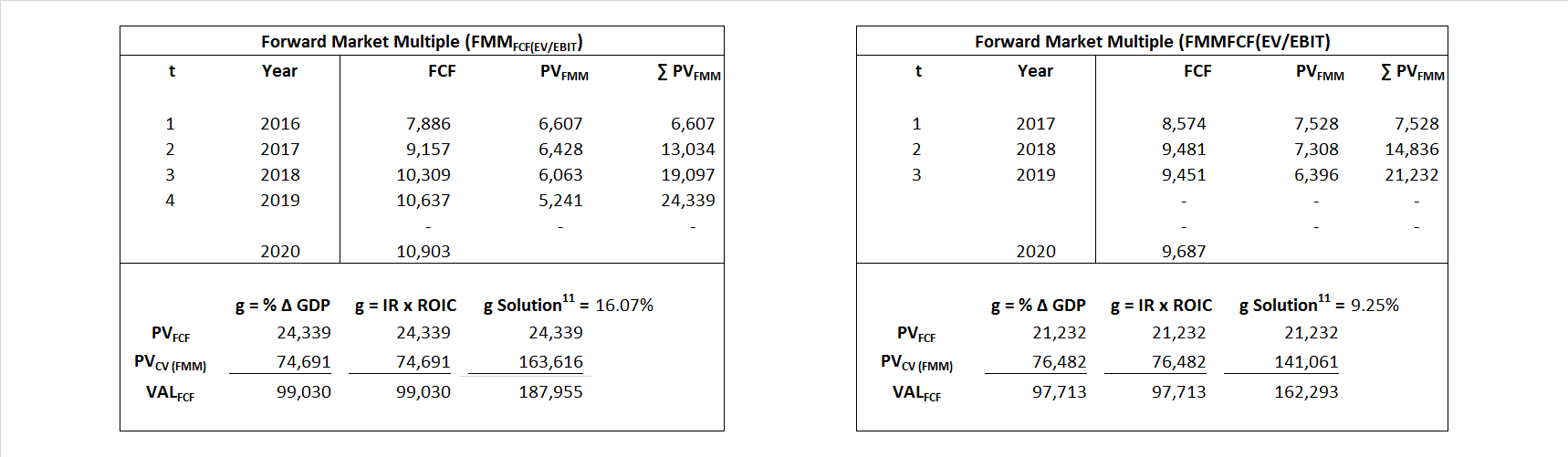# Disney

## Analyst Listing

The following analysts provide coverage for the subject firm as of May 2016:

 Broker Analyst Analyst Email JBL Advisors Jeffrey B. Logsdon jeff@jbladvisors.com Topeka Capital Markets David W. Miller dwm@topekacapitalmarkets.com Drexel Hamilton Tony Wible twible@drexelhamilton.com Evercore ISI Vijay Jayant vijay.jayant@evercoreisi.com Pivotal Research Group Brian Wieser brian@pvtl.com Guggenheim Securities Michael C. Morris michael.morris@guggenheimpartners.com RBC Capital Markets Steven Cahall steven.cahall@rbccm.com Atlantic Equities Hamiton Faber h.faber@altantic-equities.com Cowen & Company Doug Creutz doug.creutz@cowen.com Wells Fargo Securities Marci Ryvicker marci.ryvicker@wellsfargo.com Piper Jaffray Stan Meyers stan.x.meyers@pjc.com Stifel Nicolaus Benjamin E. Mogil bmogil@stifel.com FBR Capital Markets & Co Barton Crockett bcrockett@fbr.com Jefferies John Janedis jjanedis@jefferies.com Needham Laura Martin lmartin@needhamco.com BMO Capital Markets Daniel Salmon dan.salmon@bmo.com Nomura Research Anthony DiClemente anthony.diclemente@nomura.com Credit Suisse Omar Sheikh omar.sheikh@credit-suisse.com Deutsche Bank Research Bryan Kraft bryan.kraft@db.com Bernstein Research Todd Juenger todd.juenger@bernstein.com Hilliard Lyons Jeffrey S. Thomison jthomison@hilliard.com Rosenblatt Securities Martin Pyykkonen mpyykkonen@rbit.com

## Primary Input Data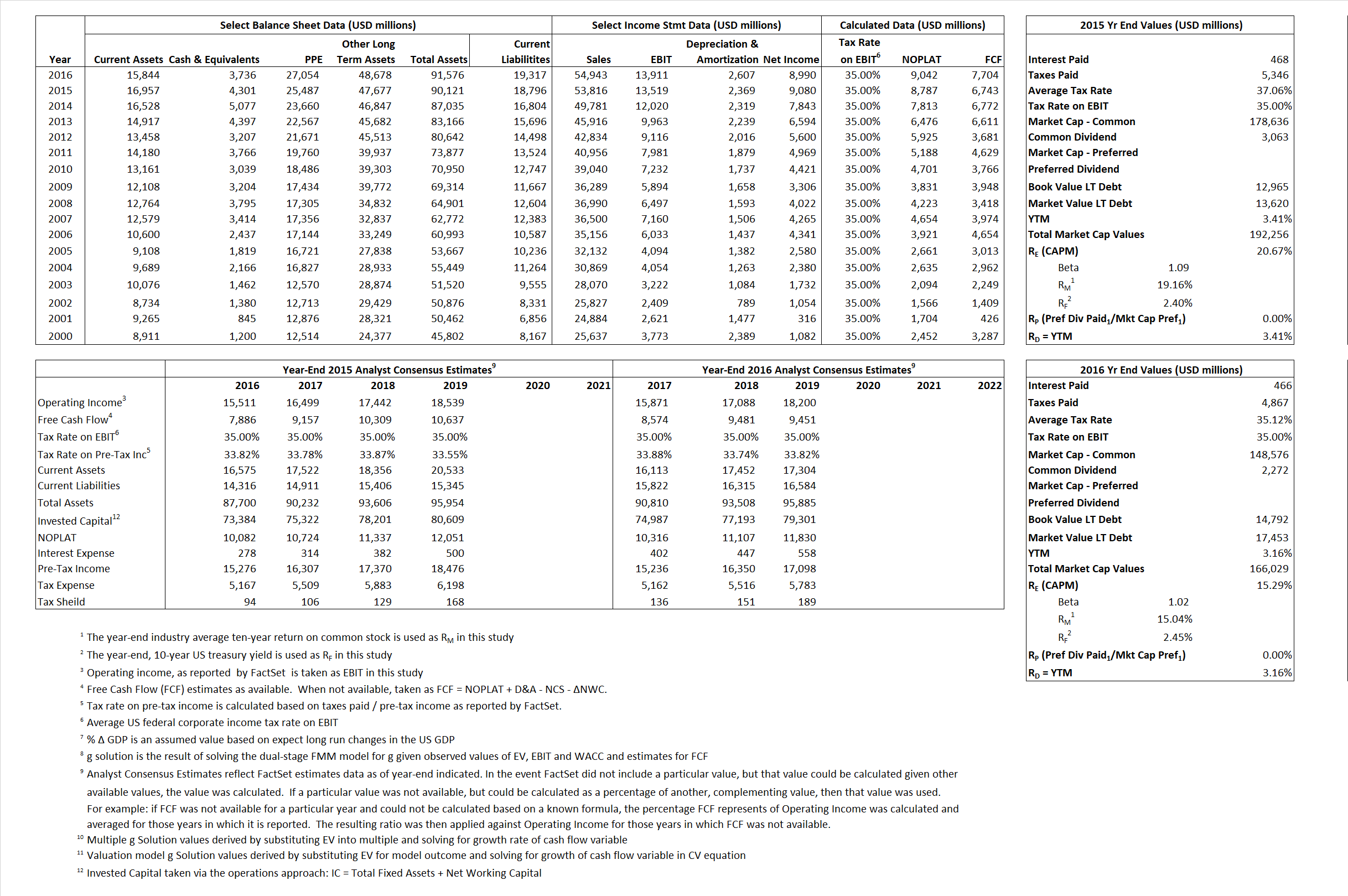## Derived Input Data

### Equational Form

Net Operating Profit Less Adjusted Taxes NOPLAT  8,787   9,042$NOPLAT\, =\, EBIT\, x\, (1 \,-\, Avg \,\,Tax\,\, Rate\,\, on\,\, EBIT)$
Free Cash Flow FCF  6,743  7,704$FCF\,=NOPLAT\,+\,Non-Cash\,Expenses-\Delta NWC\,-\,NCS$
Tax Shield TS  173  164$TS\,=\,Interest\,\,Paid\,\,x\,\, Avg \,\,Tax\,\,Rate\,\, on\,\, Pre-Tax\,\, Income$
Invested Capital IC  71,325  72,259$IC\,=\,Fixed\,\,Operating\,\,Assets\,\,+\,\,Net\,\, Working\,\, Capital$
Return on Invested Capital ROIC 12.32% 12.51%$ROIC\,=\,\frac { NOPLAT }{ IC }$
Net Investment NetInv  3,463  3,541$NetInv\,=\,{ {IC}_{1}}-{{IC}_{0}}+Depreciation$
Investment Rate IR 39.41% 39.16%$IR\,=\,\frac {NetInv}{NOPLAT}$
Weighted Average Cost of Capital WACCMarket 19.36% 13.90%$WACC\,=\,\frac { E }{ V } { R }_{ E }\,+\,\frac { P }{ V } { R }_{ P }\,+\,\frac { D }{ V } { R }_{ D }\left( 1- Avg\,\, Tax\,\,Rate\,\,on\,\,Pre-Tax\,\,Income \right)$
WACCBook  8.99% 8.55%
Enterprise value EVMarket  187,955  162,293$EV\,=\,Market\,\,Cap\,\,Equity\,+\,\,Long\,\,Term\,\,Debt\,-\,Cash$
EVBook  187,300  159,632
EV/EBIT Multiple$\frac{EV_{Market}}{EBIT}$  13.90  11.67$EV/EBIT\,=\,\frac { EV}{ EBIT}$
Long-Run Growth g = IR x ROIC
4.86%   4.90% Long-run growth rates of the income variable  are used in the Continuing Value portion of the valuation models.
g = %$\Delta$ GDP    2.50%   2.50%

## Valuation Model Outcomes

The outcomes presented in this study are the result of original input data, derived data, and synthesized inputs and, depending on the equational form of any particular valuation model, may result in irrelevant or implausible results.  For example, in the event WACC < g, the value of this term, often found in the denominator of an equation’s continuation value term, will be expressly negative and may result in a negative overall valuation for the firm.  In the event of a WACC < g relation, the model form as applied to the subject firm offers an irrelevant outcome.

### Equational form

Key Value Driver (NOPLAT) KVD (NOPLAT)${ Value }_{ DCF/KVD }=\sum { \frac { NOPLAT_{ t } }{ { \left( 1+WACC \right) }^{ t } } +\frac { \frac { { NOPLAT }_{ 1 }\left( 1-\frac { g }{ ROIC } \right) }{ WACC-g } }{ { \left( 1+WACC \right) }^{ t } } }$Key Value Driver (FCF) KVD (FCF)${ Value }_{ DCF/KVD }=\sum { \frac { FCF_{ t } }{ { \left( 1+WACC \right) }^{ t } } +\frac { \frac { { NOPLAT }_{ 1 }\left( 1-\frac { g }{ ROIC } \right) }{ WACC-g } }{ { \left( 1+WACC \right) }^{ t } } }$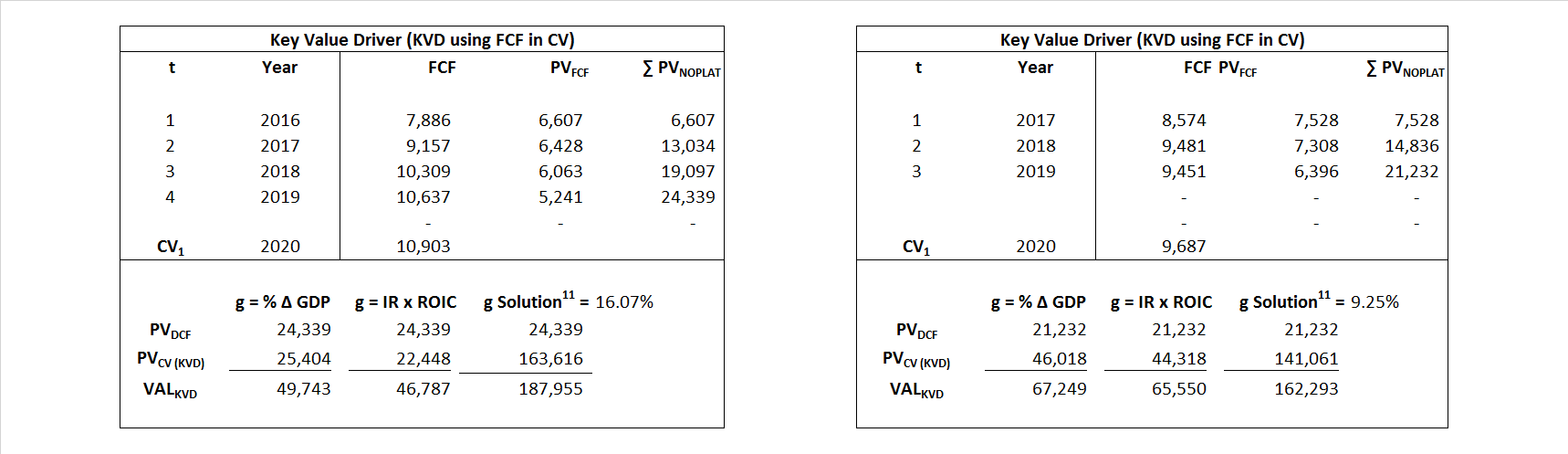Free Cash Flow FCF${ Value }_{ DCF/FCF }=\sum { \frac { FCF_{ t } }{ { \left( 1+WACC \right) }^{ t } } +\frac { \frac { { FCF }_{ 1 }}{ WACC-g } }{ { \left( 1+WACC \right) }^{ t } } }$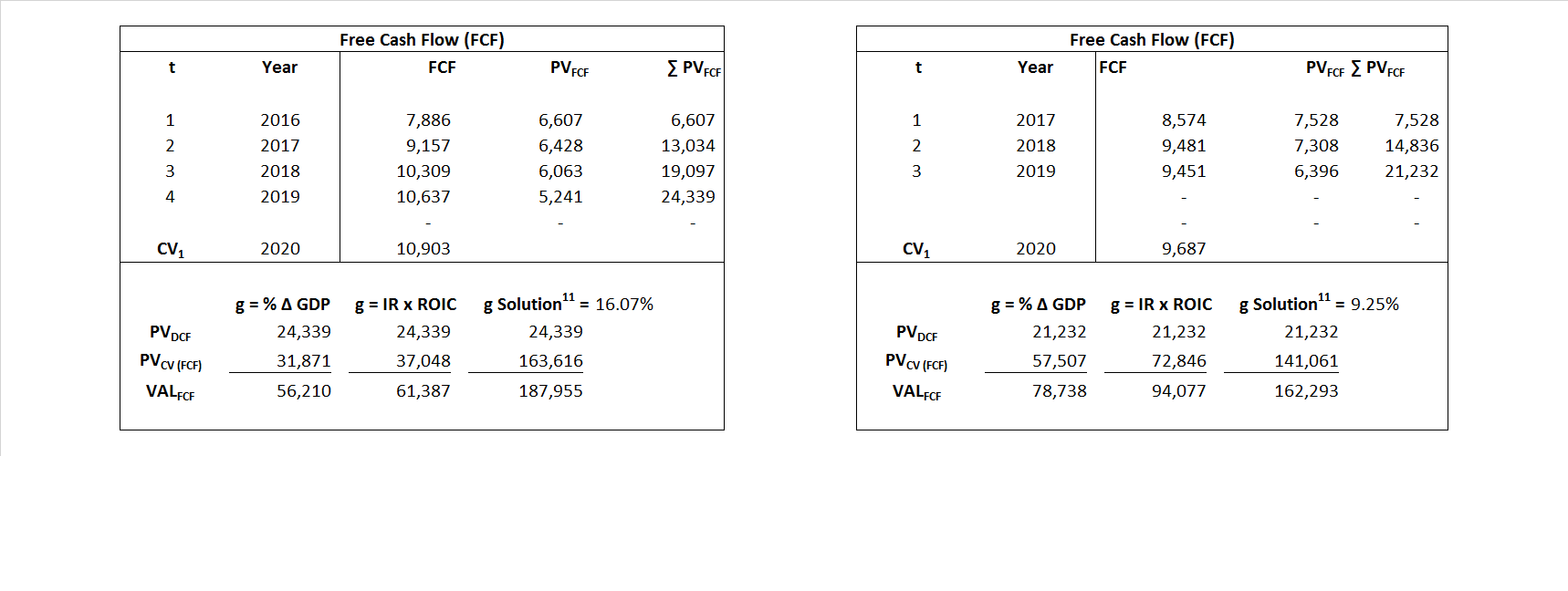Economic Profit ECON π${ Value }_{ { ECON\pi } }= I{ C }_{ 0 }+\sum { \frac { { IC }_{ t-1 }(ROI{ C }_{t}-WAC{C}_{t}) }{ { \left( 1+WACC \right) }^{ t } }+ \frac {\frac { I{C}_{0}\ x\ (ROI{C}_{1}\ -\ WAC{C}_{1}) }{ WACC-g } }{ { \left( 1+WACC \right) }^{ t } } }$Adjusted Present Value APV${ Value }_{ APV }=\sum { \frac { FCF_{ t } }{ { \left( 1+{ k }_{ u } \right) }^{ t } } +\frac { \frac { { FCF }_{ 1 }}{ { k }_{ u }-g } }{ { \left( 1+{ k }_{ u } \right) }^{ t } } } +\sum { \frac { { TS }_{ t } }{ { \left( 1+{ k }_{ tax } \right) }^{ t } } +\frac { \frac { { TS }_{ 1 }}{ { k }_{ tax }-g } }{ { \left( 1+{ k }_{ tax } \right) }^{ t } } }$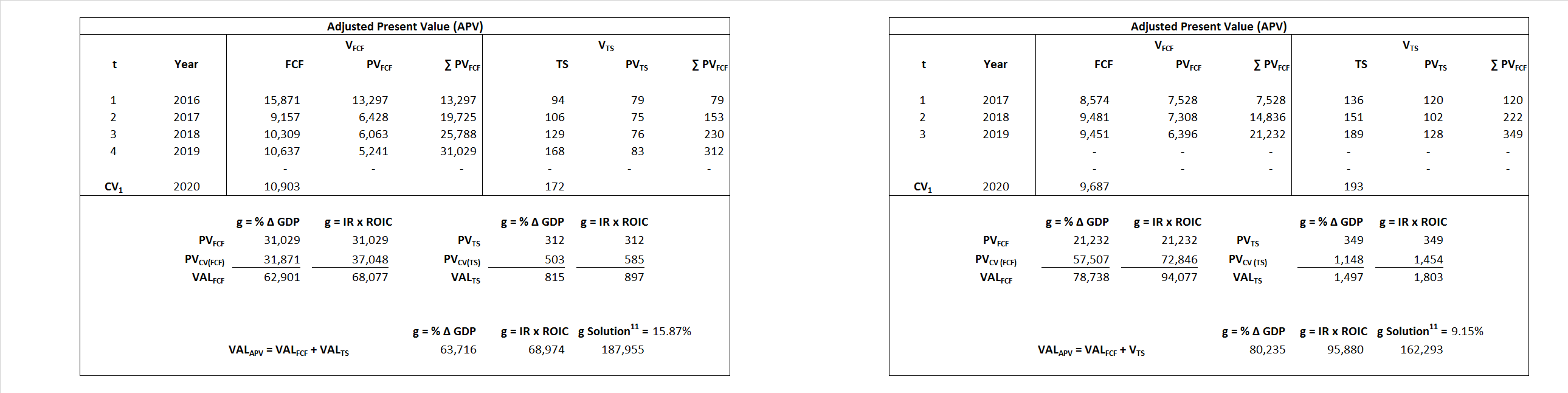Forward Market Multiple FMM${ Value }_{ DCF/FMM}=\sum { \frac { FCF_{ t } }{ { \left( 1+WACC \right) }^{ t } } +\frac { { EBIT }_{ 1 }\,{x}\,{FMM}}{ { \left( 1+WACC \right) }^{ t } } }{\,\,\,; \,\,FMM\,=\,\frac{{EV}_{t=0}}{{EBIT}_{t=0}}}$